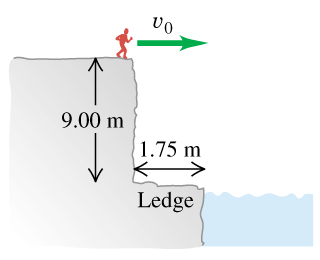# Problem: A daring 510-N swimmer dives off a cliff with a running horizontal leap, as shown in the figure .What must her minimum speed be just as she leaves the top of the cliff so that she will miss the ledge at the bottom, which is 1.75 m wide and 9.00 m below the top of the cliff?

###### FREE Expert Solution

The swimmer dives only with horizontal speed.

Therefore, v0x = v0

v0y = 0.

Let's consider the kinematic equation for the motion in the y-direction

$\overline{){\mathbf{∆}}{\mathbf{y}}{\mathbf{=}}{{\mathbf{v}}}_{{\mathbf{0}}{\mathbf{y}}}{\mathbf{t}}{\mathbf{-}}\frac{\mathbf{1}}{\mathbf{2}}{{\mathbf{gt}}}^{{\mathbf{2}}}}$

For the x-direction, we'll use:

$\overline{){\mathbf{∆}}{\mathbf{x}}{\mathbf{=}}{{\mathbf{v}}}_{{\mathbf{0}}{\mathbf{x}}}{\mathbf{t}}{\mathbf{+}}\frac{\mathbf{1}}{\mathbf{2}}{{\mathbf{at}}}^{{\mathbf{2}}}}$

To get the time, t, we'll use the equation in the y-direction.

We know that v0y = 0

79% (52 ratings)###### Problem Details

A daring 510-N swimmer dives off a cliff with a running horizontal leap, as shown in the figure.

What must her minimum speed be just as she leaves the top of the cliff so that she will miss the ledge at the bottom, which is 1.75 m wide and 9.00 m below the top of the cliff?